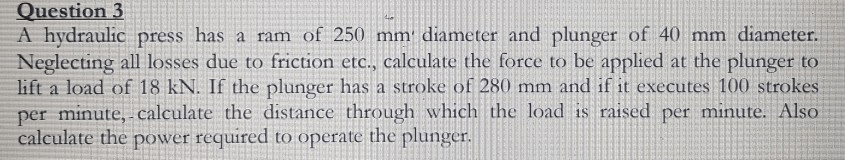# Question 3 A hydraulic press has a ram of 250 mm diameter and plunger of 40 mm diameter. Neglecting all losses due to frictio A hydraulic press has a ram of 250 mm diameter and plunger of 40 mm diameter. Neglecting all losses due to friction etc., calculate the force to be applied at the plunger to lift a load of 18 kN. If the plunger has a stroke of 280 mm and if it executes 100 strokes per minute,- calculate the distance through which the load is raised per minute. Also calculate the power required to operate the plunger.

Question-AnswerCategory: Fluid MechanicsQuestion 3 A hydraulic press has a ram of 250 mm diameter and plunger of 40 mm diameter. Neglecting all losses due to frictio A hydraulic press has a ram of 250 mm diameter and plunger of 40 mm diameter. Neglecting all losses due to friction etc., calculate the force to be applied at the plunger to lift a load of 18 kN. If the plunger has a stroke of 280 mm and if it executes 100 strokes per minute,- calculate the distance through which the load is raised per minute. Also calculate the power required to operate the plunger.A hydraulic press has a ram of 250 mm diameter and plunger of 40 mm diameter.
Neglecting all losses due to friction etc., calculate the force to be applied at the plunger to
lift a load of 18 kN. If the plunger has a stroke of 280 mm and if it executes 100 strokes
per minute,- calculate the distance through which the load is raised per minute. Also
calculate the power required to operate the plunger.